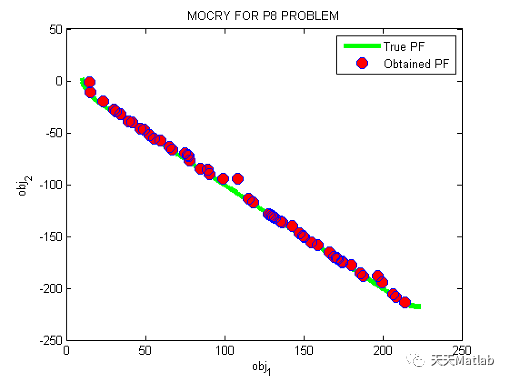# 【智能优化算法-晶体结构算法】基于晶体结构算法求解多目标优化问题附Matlab代码

+关注继续查看

## 1 内容介绍

Metaheuristics are computational procedures that intelligently lead the search process through the efficient exploration of the search space associated with an optimization problem. With the progressive outburst of problems with large data sets in various fields, there is an ongoing quest for enhancing existing metaheuristic algorithms as well as developing new ones with greater accuracy and efficiency. In general, a powerful and efficient metaheuristic algorithm is based on a rich inspiration source, implemented effectively through a precise mathematical model. Aiming to develop a highly efficient, nature-inspired optimization algorithm, here we propose a novel metaheuristic called Crystal Structure Algorithm (CryStAl). This method is chiefly inspired by the principles underlying the formation of crystal structures from the addition of the basis to the lattice points, which is a natural phenomenon that can be seen in the symmetric arrangement of constituents (i.e. atoms, molecules, or ions) in crystalline minerals such as quartz. A total number of 239 mathematical functions which are categorized into four different groups are utilized to evaluate the overall performance of the proposed method. To validate the results of this novel algorithm, 12 different classical and modern metaheuristic algorithms are selected from the literature. The minimum, mean, and standard deviation values alongside the number of function evaluations for CryStAl and the other metaheuristics for a specific tolerance are calculated and presented accordingly. The obtained results, further supported by a complete statistical analysis, demonstrated that the proposed algorithm is capable of providing very competitive results, outperforming the other metaheuristics in most cases.

## 2 仿真代码

%__________________________________________________________________ %

%

% ----------------------------------------------------------------------- %

function [occ_cell_index occ_cell_member_count]=GetOccupiedCells(pop)

GridIndices=[pop.GridIndex];

occ_cell_index=unique(GridIndices);

occ_cell_member_count=zeros(size(occ_cell_index));

m=numel(occ_cell_index);

for k=1:m

occ_cell_member_count(k)=sum(GridIndices==occ_cell_index(k));

end

end

## 3 运行结果编辑

## 4 参考文献

 Talatahari, S. , et al. "Crystal Structure Algorithm (CryStAl): A Metaheuristic Optimization Method." IEEE Access PP.99(2021):1-1.

### 博主简介：擅长智能优化算法、神经网络预测、信号处理、元胞自动机、图像处理、路径规划、无人机等多种领域的Matlab仿真，相关matlab代码问题可私信交流。

|
22天前
|

15 0
|
27天前
|

23 0
|
2月前
|

【XGBoost回归预测】基于鲸鱼算法WOA优化XGBoost实现数据回归预测附matlab代码
【XGBoost回归预测】基于鲸鱼算法WOA优化XGBoost实现数据回归预测附matlab代码
73 1
|
3月前
|

61 0
|
3月前
|

【地震】基于有限差分 (FDTD) 模拟地震超材料（晶体）时域分析附matlab代码
【地震】基于有限差分 (FDTD) 模拟地震超材料（晶体）时域分析附matlab代码
47 0
|
3月前
|

44 0
|
3月前
|

【微电网】基于麻雀算法的微电网优化调度附matlab代码
【微电网】基于麻雀算法的微电网优化调度附matlab代码
52 0
|
3月前
|

【BP回归预测】基于粒子群算法PSO优化BP神经网络实现预测多输入多输出附matlab代码
【BP回归预测】基于粒子群算法PSO优化BP神经网络实现预测多输入多输出附matlab代码
33 0
|
3月前
|

【高强度聚焦超声模拟器】模拟分层介质中的高强度聚焦超声波束和加热效应（Matlab代码）
【高强度聚焦超声模拟器】模拟分层介质中的高强度聚焦超声波束和加热效应（Matlab代码）
22 1
|
3月前
|

43 0